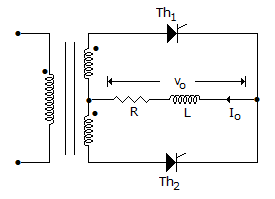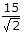# Electronics and Communication Engineering - Power Electronics

Exercise : Power Electronics - Section 1
1.
A cycloconverter can be
step down
step up
step down or step up
none of the above
Explanation:

The output frequency can be more or less than input frequency.

2.
In the below figure the average load current is 15 A. The rms value of transformer secondary current is15 A
10.61 A
7.5 A
14.14 A
Explanation:

RMS secondary current == 15/1.414 = 10.608 A.

3.
In a 3 phase fully controlled converter the firing frequency is
3 times the line frequency
6 times the line frequency
9 times the line frequency
12 times the line frequency
Explanation:

It is a 6 pulse converter.

4.
Two thyristor of same rating and same specifications
will have equal turn on and turn off periods
will have equal turn on but unequal turn off periods
may have equal or unequal turn on and turn off periods
will have unequal turn on and turn off periods